## #StackBounty: #unity #3d #rotation #raycasting Player rotation recalculation causes jittering on edges

### Bounty: 50

When my player walks around a circular planet everything works well, but when my planet is a shape like a cube or a dodecahedron, even with smoothed edges, my character jitters if I walk slowly on the edge.

That is because I raycast downward and set my characters rotation to be perpendicular with the normal. If im on the edge, the ray hits a different side of the shape than the one I stand on, causing my player to rotate to that new side, even if the angle between the face I stand on and the one I hit is 179°. So when My character rotates, the face I hit with the ray becomes the one I was standing on before, causing me to rotate back and forth between 2 faces.

Here is a beautiful scheme of what I mean: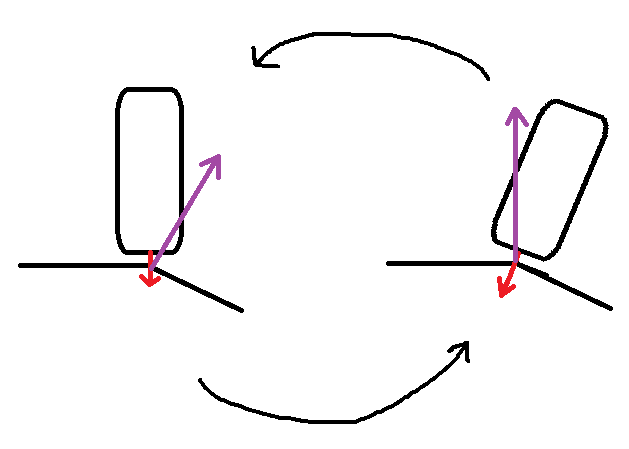I tried using SphereCast instead of Raycast, but it didn’t work. How can I limit the back and forth rotations ?

Here is the script for the raycast:

``````void Update(){
``````

`````` if (Physics.Raycast(rayStart, GetDirection(IsGrounded, GravityChangeHitPoint), out RaycastHit hit, Mathf.Infinity, layerMask))
{
Debug.DrawLine(transform.position, hit.point,Color.red);
Quaternion matchSurface;

if (GravityChangeHitPoint == GravityCenter.position)
matchSurface = PlayerLookRotation(transform.forward, hit.normal);
else
matchSurface = PlayerLookRotation(transform.forward, -GetDirection(IsGrounded, GravityChangeHitPoint));

Quaternion cam = Quaternion.Euler(0, Input.GetAxis("Mouse X") * sensitivityX, 0);

if (!IsGrounded)
transform.rotation = Quaternion.Lerp(transform.rotation, matchSurface, Time.deltaTime * damping) * cam;
else
transform.rotation = matchSurface * cam;
}
}

Quaternion PlayerLookRotation(Vector3 approximateForward, Vector3 exactUp)
{
Quaternion zToUp = Quaternion.LookRotation(exactUp, -approximateForward);
Quaternion yToZ = Quaternion.Euler(90, 0, 0);

return zToUp * yToZ;
}
``````

Get this bounty!!!

## #StackBounty: #virtualization #opengl #3d Virt-manager OpenGL (virgil) acceleration gets only 1/4 of screen

### Bounty: 50

I’m trying to use virgil OpenGL acceleration on ubuntu 20.04.2 both host and guest, but I get only 1/4 of the screen no matter what I do:It’s an intel i7 11th gen, Iris Xe GPU (Dell XPS 13)

UPDATE: as pointed out below, the problem is with scaling. How can I make this work with scaling on?

Get this bounty!!!

## #StackBounty: #3d #graphic-design Is there an external, up-to-date tool that can render scenes made in Carrara?

### Bounty: 50

Carrara is a 3D modelling tool that has the advantage of being easy to use for my purposes (creating landscapes), but the disadvantage is it has not been updated since 2013, and its unlikely, I would guess, that future updates will occur.

I’d like to continue using Carrara for creating scenes, but the rendering tool is probably 10 years out-of-date. Is there any software tool that can completely render a scene created in Carrara, but that is up-to-date, so takes advantages of newer ways to render?

Get this bounty!!!

## #StackBounty: #unity #3d #animation Strange animation behavior for my 3D model

### Bounty: 100

On my Unity project I have a strange behavior for my 3D model which I bought on the Unity asset store.

If I move the player, there appears a wired shine / glow… seems that the character does not move completely, but drags…

Here a screenshot to show you the behavior:Here is a video where you can see that the animations are not clean: https://youtu.be/3H3r6HcgSaQ

Here you can see the configuration of my camera and PostProcessingVolume:

By the way: I’m using the Built In Rendering Pipeline. This appears not only on this 3d model. I can see this behavior for also other 3d models in my scene.

What could be the issue here? I have no idea…

Get this bounty!!!

## #StackBounty: #android #3d #augmented-reality #arcore How can i scan a qr code and place an 3d object using ar core?

### Bounty: 100

here i need some suggestion or a want to a way of doing this

scenario : i want to scan a qr code and after scanning the qr code i want place a 3d object in the ar scene . here i dont want to use google vision api and instead i want to use any qr reader package and want to place it. is there reference / url so that it will help me to achieve this ?

i used this package for qr scan https://github.com/zxing/zxing

Get this bounty!!!

## #StackBounty: #android #android-studio #3d #arcore #sceneform Draw Renderable Shape on 3D Model using Sceneform Android

### Bounty: 50

I am using SceneForm Android SDK to render 3D Model in Android APP.

To display the 3D Model I am using this code below which also supports Rotating and Zooming feature in 3D Model

`````` private fun renderLocalObject(position: Int) {

ModelRenderable.builder()
.setSource(appCompatActivity, Uri.parse(models.get(position)))
.setRegistryId(models.get(position))
.build()
.thenAccept { modelRenderable: ModelRenderable ->
}
.exceptionally { throwable: Throwable? ->
var message: String?
message = if (throwable is CompletionException) {
"Internet is not working"
} else {

}
null
}
}

if (sceneView != null) {

val transformationSystem = makeTransformationSystem()
val dragTransformableNode = DragTransformableNode(5f, transformationSystem)
dragTransformableNode.renderable = model
dragTransformableNode.select()

sceneView!!.getScene()
.addOnPeekTouchListener { hitTestResult: HitTestResult?, motionEvent: MotionEvent? ->
transformationSystem.onTouch(
hitTestResult,
motionEvent
)

when (motionEvent?.action) {
MotionEvent.ACTION_UP ->
if (hitTestResult != null) {
}

}

}
}
}
``````

I want to draw a sphere renderable shape directly over the 3d model, but the Node is not getting stuck model.

Below is the required output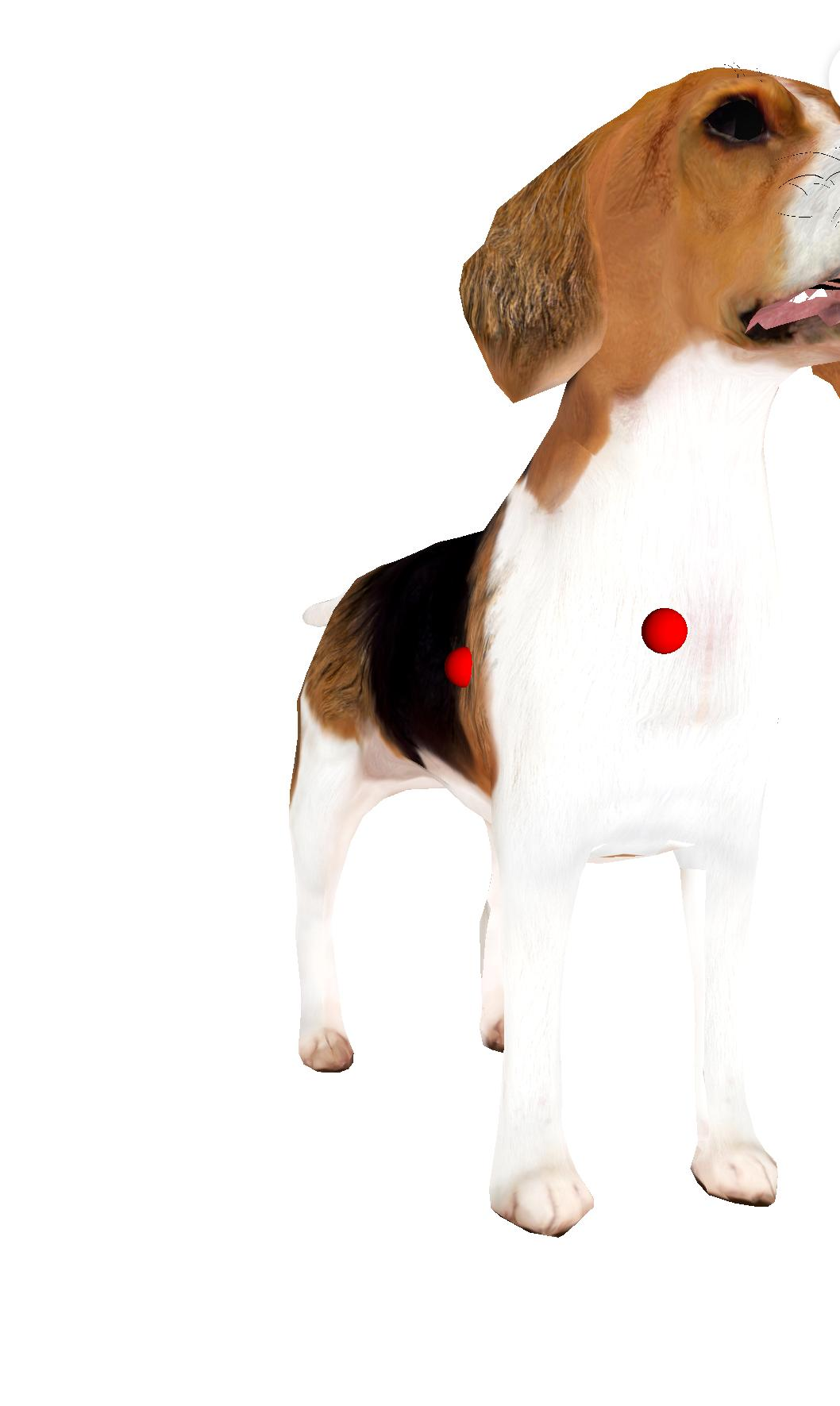Below is the final output of my code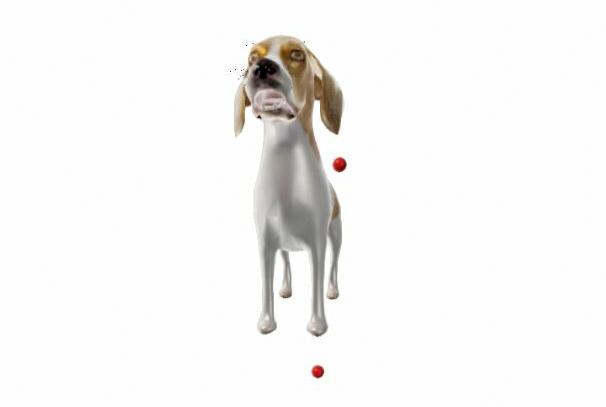This is my code for adding node

``````  private fun addDot(hitTestResult: HitTestResult){
val color = Color(.8f, 0f, 0f)
MaterialFactory.makeOpaqueWithColor(appCompatActivity, color)
.thenAccept { material->
// The sphere is in local coordinate space, so make the center 0,0,0
val sphere = ShapeFactory.makeSphere(0.05f, Vector3.zero(),
material)
val indicatorModel = Node()
indicatorModel.setParent(hitTestResult.node)
indicatorModel.worldPosition = hitTestResult.point
//  indicatorModel.localPosition = Vector3(0f, 0f, -1f)
indicatorModel.renderable = sphere

}
}
``````

Is there any way to draw shape directly over the 3d model.

Get this bounty!!!

## #StackBounty: #javascript #math #graph #3d #2d JavaScript 3D Chart Library for Math/Engineering. Projection of 3D figures to 2D

### Bounty: 100

I’m looking for a JavaScript library for plotting 3D charts with figures like Cylinder, Box, Torus, Sphere etc for mathematical/engineering purposes.

The library I’m looking for should have ability to mark some important sections of the graph like function name, intersections, crossing points etc.

There is no need for any 3D shading to show a shape of a 3D figure. Instead the library should draw dashed lines in case they are covered by the front of a 3D figure.

I found a good 2D library for drawing geometry JSX Graph that is well suited for the purpose but unlucky it lacks drawing 2D projection of 3D figures.

Below some examples from JSX Graph to picture some of the features that are required.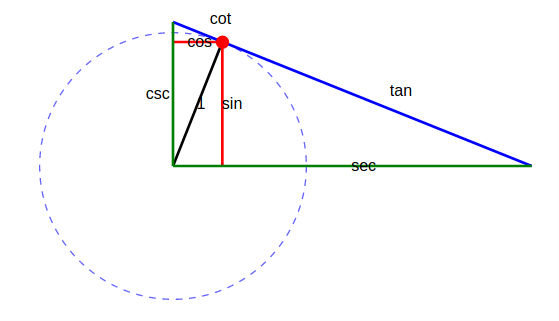Adding auxiliary lines as dashed, adding labels to graphs, marking an important joint point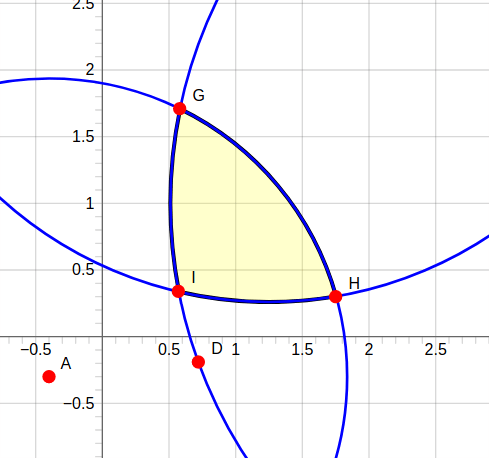Adding points of lines’ cross, marking an intersection area.

In short the library should be able to make 3D graphs like these you can find in books or a technical documentation.
Do you know any library like JSX graph but with a support of projecting 3D figures to the 2D?

Get this bounty!!!

## #StackBounty: #3d #computational-geometry #mesh #geometry #cad Algorithm to select regions based on curvature on a mesh

### Bounty: 50

I’m trying to understand how to implement an algorithm similar to the one used by Magics’ mark surface tool, you can see such behaviour on this video.

Quoting the video: "Basically with this tool you’re able to select surfaces, which unlike planes, surfaces take curvature into account."

The first idea that come to my mind to implement something similar was starting by considering the adjacency information of the mesh and consider on the computation the angle adjacent triangle normals. My idea was that if such an angle wasn’t on the range [pi/2-tol, pi/2+tol] two adjacent triangles would be "smooth". This thought was too naive and the idea would just work for a very limited of cases and it’d start fail for many of them.

After that, I’ve spent a little bit of time reading some papers talking about mesh segmentation and it seems this has been an area of research for many years… But before even considering implementing any of these one I’d like to ask here if you knew some basic&good enough algorithm I could implement that could behave in a similar fashion to Magic’s.

So yeah, that’s my question basically, assuming a triangular mesh that has adjacency information built (ie: you can check adjacent triangles from any given face) and a starting selected triangle, how would you detect the "surface" region associated to it?

Get this bounty!!!

## #StackBounty: #tikz-pgf #diagrams #3d #tikz-cd #tikz-3d Deforming/projecting text in TikZ(-cd) along a curved surface

### Bounty: 50

This is a continuation of STeX Exchange 552946.

I’ve been trying to get some 3D effects on tikz-cd, and got an amazing answer by @ZhiyuanLck. The only remaining problem is getting text/arrows to be drawn along curved surfaces (or look that way). Is it possible to achieve such an effect?

For instance, how would we do that for the `Rightarrow`s and their labels `theta_f` and `theta_g` in the diagram below?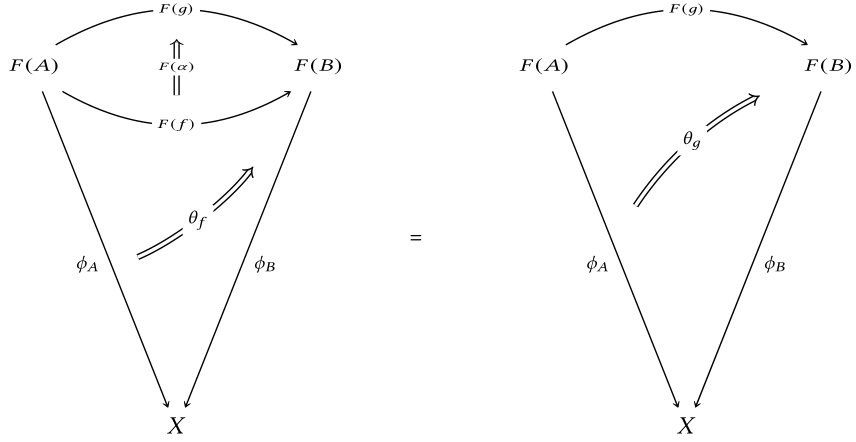(This diagram is the ice cream cone condition for lax slice bicategories, as in Section 7.1 of Johnson–Yau’s new book on bicategories.)

Compilable code for this diagram:

``````documentclass[english,11pt]{standalone}
RequirePackage{luatex85}
usepackage{tikz}
usepackage{tikz-cd}
usetikzlibrary{3d}
makeatletter
tikzset{
plane/.code args={#1and#2}{
tikz@scan@one@pointpgf@process#1
edeftemp@a{(thepgf@x, thepgf@y)};
tikz@scan@one@pointpgf@process#2
edeftemp@b{(thepgf@x, thepgf@y)};
pgfkeysalso{
plane x={temp@a},
plane y={temp@b},
canvas is plane,
}
},
}
makeatother
usepackage{libertine}
usepackage{mathtools}
usepackage[libertine]{newtxmath}
tikzcdset{
arrow style=tikz,
%diagrams={>={Straight Barb[scale=1.5]}}
diagrams={>={Stealth[round,length=4pt,width=4.95pt,inset=2.75pt]}}
}
begin{document}
newsavebox{BoxNodeOne}
savebox{BoxNodeOne}{
begin{tikzcd}[row sep={4.5em,between origins}, column sep={4.5em,between origins}, ampersand replacement=&]
{}
arrow[r, "F(A)"{plane={(1,0) and (0,0.7)}},phantom]
&
{}
end{tikzcd}
}
newsavebox{BoxNodeTwo}
savebox{BoxNodeTwo}{
begin{tikzcd}[row sep={4.5em,between origins}, column sep={4.5em,between origins}, ampersand replacement=&]
{}
arrow[r, "F(B)"{plane={(1,0) and (0,0.7)}},phantom]
&
{}
end{tikzcd}
}
newsavebox{BoxOne}
savebox{BoxOne}{
begin{tikzcd}[row sep={4.5em,between origins}, column sep={4.5em,between origins}, ampersand replacement=&]
{}
\
arrow[u, Rightarrow]
{}
end{tikzcd}
}
newsavebox{BoxTwo}
savebox{BoxTwo}{
begin{tikzcd}[row sep={3.6em,between origins}, column sep={3.6em,between origins}, ampersand replacement=&]
{}
&
{}
\
{}
arrow[ru, Rightarrow, bend right=35]
&
{}
end{tikzcd}
}
begin{tikzcd}[row sep={14.4em,between origins}, column sep={6.3em,between origins}, ampersand replacement=&]
{hspace{+1.25em}usebox{BoxNodeOne}}
arrow[rr, "F(g)"{name=3,description,plane={(1,0) and (0,0.7)}},bend left=30]
arrow[rr, "F(f)"{name=2,description,plane={(1,0) and (0,0.7)}},bend right=30]
arrow[rd, "phi_{A}"'{name=1},start anchor={[xshift=+0.5em]}]
&
&
{hspace{-1.25em}usebox{BoxNodeTwo}}
arrow[ld, "phi_{B}",start anchor={[xshift=-0.5em]}]
\
{}
&
X
&
{}
% 2-Arrows
arrow[from=1,to=1-3,"theta_{f}"{description,yshift=-0.2em},shorten=2.5em,Rightarrow,xshift=-1.0em,yshift=-1.0em,bend right=15]
arrow[from=2,to=3,"usebox{BoxOne}"{plane={(1,0) and (0,0.6)}},shorten=0.5em,phantom]
arrow[from=2,to=3,"scalebox{0.75}{\$F(alpha)\$}"{description,plane={(1,0) and (0,0.6)}},shorten=0.5em,phantom]
end{tikzcd}
=
begin{tikzcd}[row sep={14.4em,between origins}, column sep={6.3em,between origins}, ampersand replacement=&]
{hspace{+1.25em}usebox{BoxNodeOne}}
arrow[rr, "F(g)"{name=3,description,plane={(1,0) and (0,0.7)}},bend left=30]
arrow[rd, "phi_{A}"'{name=1},start anchor={[xshift=+0.5em]}]
&
&
{hspace{-1.25em}usebox{BoxNodeTwo}}
arrow[ld, "phi_{B}",start anchor={[xshift=-0.5em]}]
\
&
X
&
% 2-Arrows
arrow[from=1,to=1-3,"theta_{g}"{description,yshift=+0.15em},shorten=1.5em,Rightarrow,bend left=15,xshift=+0.25em,yshift=0.5em]
end{tikzcd}
end{document}
``````

Get this bounty!!!

## #StackBounty: #3d Showing planes in 3D

### Bounty: 150

I have a GeoGebra diagram but I want to make a Tikz picture out of it. My goal is to make the faded green planes. The vector w-v is on the plane and m is on a plane that is parallel to that green plane as well.

So far, I have a 3D tikz picture and I’ve been experimenting with the best way to make this image. In my code, I have the vectors w, v, and w-v. (The vectors w and v are perpendicular, and w-v would lie on the lower green plane.) I’ve yet to put in vectors x, m, and y, and the plane that goes through m.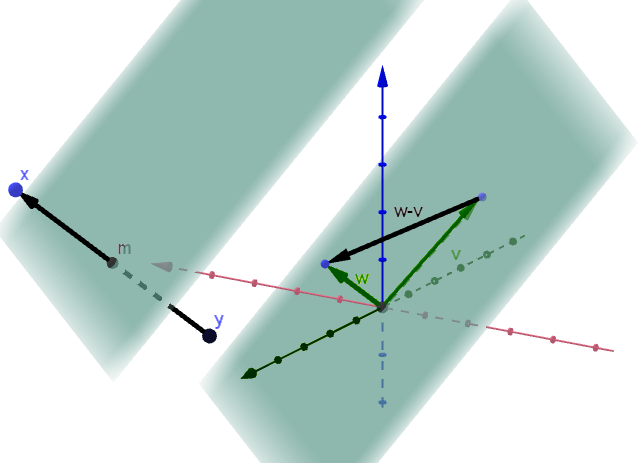``````documentclass[t]{beamer}
usepackage[utf8]{inputenc}
useoutertheme[subsection=false]{miniframes}
usepackage{pgfplots}
usepackage{amssymb}
pgfplotsset{compat=1.16,width=10cm}
usetikzlibrary{3d}
usetikzlibrary{calc}
begin{document}

begin{frame}
begin{center}
begin{tikzpicture} [x={(-0.6cm,-0.4cm)}, y={(1cm,0cm)}, z={(0cm,1cm)}, scale=1]
begin{scope}[canvas is zy plane at x=0]
fill[red, opacity = 0.1] (0, 0) rectangle (5, 5);
draw[gray!40] (0, 0) grid (5, 5);
end{scope}
begin{scope}[canvas is zx plane at y=0]
fill[green, opacity = 0.1] (0, 0) rectangle (5, 5);
draw[gray!40] (0, 0) grid (5, 5);
draw[black, -latex] (0, 0) -- (5.5, 0) node[left] {\$z\$};
draw[gray, -latex] (0, 0) -- (-0.5, 0);
foreach z in {1,...,5} draw[] (z, -0.1) -- (z, 0.1) node[left]{z};
foreach z in {-1,...,-1} draw[gray] (z, -0.1) -- (z, 0.1) node[left]{z};
end{scope}
begin{scope}[canvas is yx plane at z=0]
fill[blue, opacity = 0.1] (0, 0) rectangle (5, 5);
draw[gray!40] (0, 0) grid (5, 5);
draw[black, -latex] (0, 0) -- (5.5, 0) node[above] {\$y\$};
draw[black, -latex] (0, 0) -- (0, 5.5) node[above] {\$x\$};
draw[gray, -latex] (0, 0) -- (0, -0.5);
foreach x in {0,...,5} draw[] (-0.1, x) -- (0.1, x) node[right]{x};
foreach x in {-1,...,-1} draw[gray] (-0.1, x) -- (0.1, x) node[right]{x};
draw[gray, -latex] (0, 0) -- (-0.5, 0);
foreach y in {1,...,5} draw[] (y, -0.1) -- (y, 0.1) node[below]{y};
foreach y in {-1,...,-1} draw[gray] (y, -0.1) -- (y, 0.1) node[below]{-y};
end{scope}
%
draw[red, thick] (0, 2, 0) -- (-1, 2, 0);
draw[blue, thick] (-1, 2, 0) -- (-1, 2, 2);
draw[green, thick] (-1, 0, 0) -- (-1, 2, 0);
draw[black, ultra thick, ->] (0,0,0) -- (-1,2,2) node[below right]{\$mathbf{v}\$};
%
draw[red, thick] (0, 1, 0) -- (4, 1, 0);
draw[blue, thick] (4, 1, 0) -- (4, 1, 1);
draw[green, thick] (4, 0, 0) -- (4, 1, 0);
draw[black, ultra thick, ->] (0,0,0) -- (4,1,1) node[below left]{\$mathbf{w}\$};
%
draw[black, ultra thick, ->] (-1,2,2) -- (4,1,1);
draw[black] (2.5,1.5,1.5) node[above left]{\$mathbf{w-v}\$};
end{tikzpicture}
end{center}
end{frame}
end{document}
``````

Get this bounty!!!